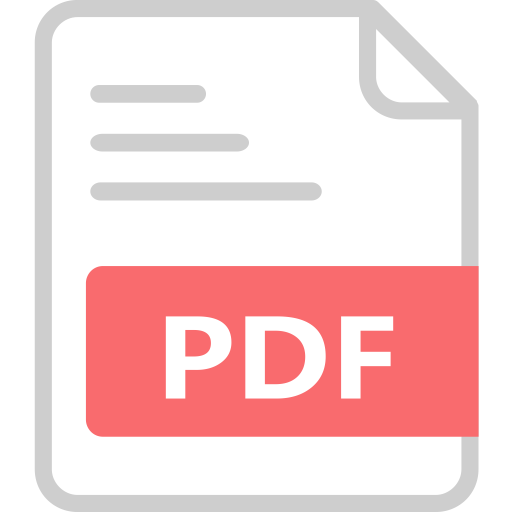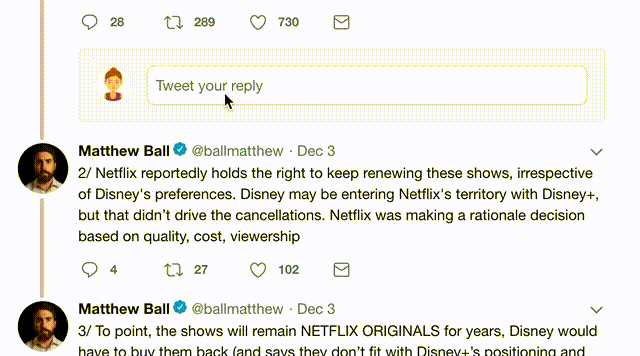, 26 tweets, 11 min read
On equations, identities, and #WhoSaysMath. Come for the pedagogy, stay for basic category theory. Thread here inspired the below.

TLDR: We can say "it's wrong" less and "it depends" more. (1/25)

#MTBoS @CmonMattTHINK @j_lanier @katemath @mrdardy
The first issue this raises is our expert blind spots. Using & teaching algebra can make us too comfortable with the meaning of an equals sign! Consider these two: (2/25)Most math teachers would identify the first line as an equation and the second as an identity.

Why? Because the first is "sometimes true" (only if x is 1) and the second is "always true." The first is a problem to solve (when is it true?) and the second a fact we can use. (3/25)But how does a student, struggling to make meaning with algebra, know the difference in meaning when they read these in their textbook? See them written on the whiteboard?

Algebra curriculum conditions novices to see "=" only in the first sense (a problem to be solved). (4/25)Mathematicians pride themselves on efficiency of communication. We have to do better with "equals." This morning's thread was about the difference below.

An equation asserts the equal value of two expressions.
An identity asserts the equality of two FUNCTIONS. (5/25)So our more specific expert blind spot is that we often elide the difference between an algebraic expression, and a function defined using that expression.

We train students to see that difference, in part, by inviting them to see f,g,h below all as "the same rule." (6/25)But functions exceed expressions, are richer than rules. Functions are relations on *sets.*

Indeed we cannot define the concept of a function without specifying the sets from which its "(input,output)" pairs are drawn. (7/25)The domain of a function is an essential part of its nature. Change the domain, and you change the function.

Even if the same expression defines both! This f is invertible; this g is not. So they cannot be "the same" function. (8/25)Many algebra textbooks ask students "find THE domain" of a function, or (worse) an expression.

Our expert blind spot: "Of course this means the MAXIMAL set of definition, so there's a right answer."

Reality: Unless it is tacit knowledge, 👏 domain 👏 is 👏 a choice 👏. (9/25)
I know: It's cumbersome to specify domains of functions where they "should be obvious."

They're tacit knowledge to us who teach & use algebra daily. But only our most privileged & high-achieving students have fluency in "Mathematish." (10/25) researchgate.net/profile/Hakan_…
Even worse for students is when we write a NUMBER and mean a function! @j_lanier 's example from this morning is a good one: is this an equation, or an identity? (11/25)Treating this as an equation invites a problem to be solved: "For what x are both sides the same number?" The answer: only when x is 0.

But is it an identity? We can't decide until you/me/someone(!) says what are the domains of the functions on both sides! #WhoSaysMath (12/25)I think @VictorPiercey1 said:
"The best math problems all have the same solution: 'It depends.'"

Is this an identity? It depends on domain chosen for f(x)=√x + √-x, and for g(x)=0. The former must be a subset of the maximal domain {0}. Latter cld be any subset of ℝ. (13/25)So, my last pedagogy point:
Always call a choice a choice. Too much dehumanization & anxiety arises in the math classroom from overuse of "THE" where "A" is appropriate. Give students agency to choose & knowledge to assess ramifications of their choice. (14/25)
If you've made it this far, maybe you came for the category theory. Don't worry, this won't hurt. I hope you'll find it accessible, even. I'm not an expert, but you'll find many on #MathTwitter who are. (15/25)
We tend to think of math as a study of mathematical constructs, objects. But usually it's more than that: we study the objects themselves, yes, but also relationships/connections/comparisons among them.

Category theory is a way to formalize that idea. (16/25)
A category consists of a collection of things (OBJECTS) and arrows (MORPHISMS) drawn among the things.

Common-sense asks: every object has an arrow to itself, we can follow two consecutive arrows to create a third, and following 3 consecutive arrows obeys associativity. (17/25)
So, in a way, high-school algebra studies the category whose objects are subsets of ℝ, and whose morphisms are functions whose domain/codomain are each one such subset.

Or maybe only the polynomial functions (algebra 1), or differentiable functions (calculus)... (18/25)
One of the threshold concepts in category theory is: really there "are no objects, only morphisms." The arrows are what really matter.

Why? Because every object has a (unique) arrow to itself, the so-called identity morphism 1ₓ : X → X. View these as "the objects!" (19/25)
ANYWAYS, with categorical perspective on "high-school algebra," you can see how even writing
f(x) = x
doesn't tell us nearly enough about f. But it does, the moment you tell me its domain. Because it "is" its domain, in a unique way! (20/25)
So the categorical perspective exposes the root of these student-favorite misapprehensions. They're not "wrong!" They're right, so long as you say WHICH IDENTITY FUNCTION is understood on the right-hand sides! (21/25)Constants? In the category whose objects = subsets of ℝ, singleton sets {a} are "terminal objects." Given a subset X ⊂ ℝ, there's a unique function cₐ : X → {a}.

That's the role of "numbers" in our category. They're (bunches of) functions too, 1 for each subset X! (22/25)
So the categorical perspective also provides a way to reconcile this favorite "error." It's an identity WHEN what we mean by "4" is "the constant function into {4} whose domain is {x : x≠2}." (23/25)More seriously, if you want to learn more about category theory, @math3ma is one of the best communicators on the subject (and many others) I've read. Here's her blog on the topic — read from bottom to top. math3ma.com/categories/cat… (25/25)
Missing some Tweet in this thread? You can try to force a refresh.

Keep Current with Matt Salomone

Stay in touch and get notified when new unrolls are available from this author!

This Thread may be Removed Anytime!Twitter may remove this content at anytime, convert it as a PDF, save and print for later use!

# Try unrolling a thread yourself!2) Go to a Twitter thread (series of Tweets by the same owner) and mention us with a keyword "unroll" `@threadreaderapp unroll`